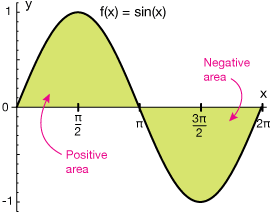# Area Under curve handwritten Notes + Previous year Questions for Jee mains 2020

Share with friends

SYLLABUS – Integral as an anti – derivative. Fundamental integrals involving algebraic, trigonometric, exponential and logarithmic functions. Integration by substitution, by parts and by partial fractions. Integration using trigonometric identities.

Integral as limit of a sum. Fundamental Theorem of Calculus. Properties of definite integrals. Evaluation of definite integrals, determining areas of the regions bounded by simple curves in standard form.

Important Topics for JEE Main 2020 in Mathematics

In the Mathematics section of JEE Main, there is an even distribution of marks for all the chapters. There are 15 chapters and all are equally important chapters for JEE Main. So, you can prioritise focusing on particular chapters which are easy and the chapters in which you have a good grip.

Below is a list of scoring chapters and important chapters for JEE Main in Mathematics:

• Sequence & Series
• Determinants and Matrices
• Sets and relations
• Mathematical Reasoning
• Statistics & Probability
• Vector & 3D
• Straight line
• Circle
• Definite Integral
• Indefinite Integral
• Application of Derivatives
• Differential equation
• Application of Integrals
• Trigonometric Ratios

MATHS EASY AND IMPORTANT CHAPTERS ( JEE MAINS 2020 )

1. Statistics- 4 marks
2. Height and Distance- 4 marks
3. Mathematical Reasonong- 4marks
4. Matrices and Determinant- 8 marks
5. Sets,Relation and function – 8 marks
6. Vector and 3D– 8 marks